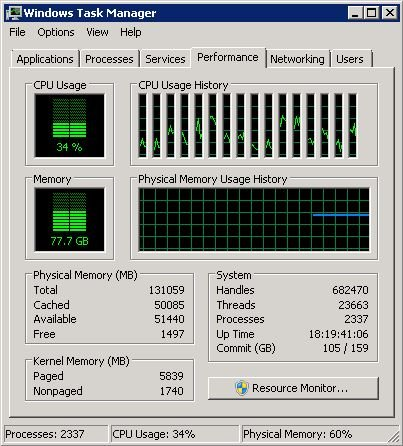Some years back, HP suggested an approach to calculate the required number of LG for a LoadRunner test. The same approach has been formulated in the below-given calculator. As the Load Generator calculator requires some inputs so it leads to run some small tests before getting the number of required LG for the test. Follow the below steps to get the input values:

1. First VUser Memory: “First VUser Memory” is the memory consumed by one VUser. To determine this value check the available memory on the LG and note it down. Then, execute a  test with 1 VUser for 15 minutes. There must be some decrease in available memory. Note down the difference between currently available memory and initially available memory. Example: Before starting the test the available memory was 51440 MB memory. The available memory reduced to 51428 MB during the test. So, the First VUser Memory value is 12 MB (=51440-51428).
2. Each Additional VUser Memory: Run the same test for 5 VUsers. Keep the same delay at the start and have each user start one minute apart. Monitor the load generators available MB of RAM and note the drop in free RAM space as each user starts. Average the amount of RAM that the second virtual user on up uses and this is the “Each Additional VUser Memory”. Example: The initially available memory was 51440 MB. During the test, it reduced to 51428 MB, 51418 MB, 51409 MB, 51399 MB and 51388 MB. Hence the average of differences is  10.4 MB (=(12+10+9+10+11)/5).
3. Total LG RAM: Determine the total RAM on the load generator. Example: 131059 MB
4. LG RAM used by OS: Determine the RAM used by Operating System on the load generator. Example: 79564 MB

### How to check RAM usage on LG?

• Right click on Task Bar of LG
• Left click on “Start Task Manager”
• “Windows Task Manager” window will open
• Click on “Performance” tab
• Under the ‘Physical Memory’ section ‘Total’ attribute will provide the size of RAM. Refer below screenshot, the total memory is 131059 MB or 128 GB.
• ‘Memory’ section shows the amount of Memory which computer is currently using. In the screenshot, it is 77.7 GB (Make sure LG should be idle so that the correct value can be captured)One of the LoadRunner community articles recommends that to calculate the number of VUsers on one load injectors is a good practice rather than go blindly and ruin the test. The statement is:

In previous versions of LoadRunner and Performance Center, a protocol footprint spreadsheet was distributed by HP to provide a guideline for how much memory a script of a particular protocol would consume. The problem was recipients of the footprint spreadsheet thought of it as definitive rather than prescriptive. It is much better to use a repeatable process and a formula to mathematically estimate the total number of users a load generator can run on a per-script basis.

## Example:

• First VUser Memory (in MB): 12
• Each Additional VUser Memory (in MB): 10.4
• Total LG RAM (in MB): 131059
• LG RAM used by OS (in MB): 79564

The calculated number of VUsers on 1 LG is 3713. Now, calculate the number of LG required to run 4500 VUsers.

• No. of VUsers in the test: 4500

The answer is 2 (Rounded-off to the higher side 1.21). Hence 2 identical LGs are required to carry out the test with 4500 VUses.

### 2 Responses

1.Rajan says:

Thanks for such an insight to this topic.

Appreciate if you can share the formula behind calculation.

the logic i undrstood is the remaining memory(Total LG RAM – LG RAM used by OS) will be allocated to the Vusers. if 1 Vuser consumes 12 MB, then (remaining memory/12 = no of Vusers which can use this remaning memory for execution). how do i found that calcualted users are correct?

Warmly,

•PerfMatrix says:

Hi Rajan,

The calculated Vuser would be a predicted value which could be changed if some other programs run on the LG at the same time (i.e. during test execution).

To verify the correctness of the formula, you can first calculate the Vuser count on the LG and try to run the same number of Vuser test on that machine (LG).